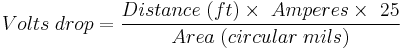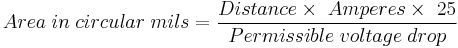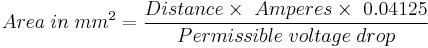# 901.12 Electrical Components

## 901.12.1 Voltage Drop and Wire Sizes

Voltage drop is an important factor in determining wire sizes. The voltage drop in any electrical circuit is directly dependent upon current and wire resistance. According to Ohm's Law the voltage drop in a line is equal to the current in amperes multiplied by the resistance of the line in ohms:

E = I x R

Where:

E = voltage

I = Current (in amperes)

R = Resistance (in ohms)

Since the resistance of a wire conductor is inversely proportional to the cross-sectional area of the wire, increased voltage drops are developed as the wire diameters decrease.

The areas of wires in circular mils (mm2) and the resistance in ohms per 1000 ft. (1000 m) to be used in calculations are shown in the tables of EPG 901.10.3 Cable and Conduit. The voltage drop in any line is calculated from the formula:

ENGLISH$Volts\; drop=\frac{Distance\;(ft) \times \ Amperes \times \ 25}{Area\;(circular\;mils)}$
METRIC$Volts\; drop=\frac{Distance\;(m) \times \ Amperes \times \ 0.04125}{Area\;(mm^2)}$

Transposing this formula, the required area in circular mils (mm2) can be computed:

ENGLISH$Area\; in\; circular\; mils=\frac{Distance \times \ Amperes \times \ 25}{Permissible\;voltage\; drop}$
METRIC$Area\; in\; mm^2=\frac{Distance \times \ Amperes \times \ 0.04125}{Permissible\;voltage\; drop}$

With the constant wattage ballast, the permissible voltage drop is five percent of the system voltage. A factor of 0.95 is to be applied to the system voltage to allow for line fluctuations. This results in an allowable drop of 23 volts for a 480-volt circuit, and 11 volts for a 240-volt circuit.

901.12.1.1 Maximum Wire Sizes. Maximum wire sizes for lighting branch circuits (cables between control station and light poles) are as follows:

• Standard Light Poles (30 and 45 ft. mounting height) and Underpass Luminaires - #6 AWG (16 mm2) MAXIMUM
• High Mast Light Poles - #2 AWG (35 mm2) MAXIMUM

If circuit loading or voltage drop requires a larger wire size than the maximum then one or more of the following can be considered:

• Increase the system voltage from 240 volts to 480 volts.
• Split the circuits into additional branch circuits. This may require parallel cable conduits in some parts of the system.
• Locate the control station closer to the lighting system.

Availability and proximity of power sources and system voltages is considered in obtaining the best solution. Where alternate configurations can achieve the same results, a rough cost comparison is made to determine the most economical configuration.

Wire sizes larger than #0 AWG (70 mm2) are not recommended for power supply cables (cables between power supply and base-mounted control station) since larger wire sizes are difficult to handle in the control stations and power supplies. If a larger wire size is required, a terminal strip is provided in the control cabinet to reduce the wire size.

901.12.1.2 Example to Determine Necessary Wire Cable Size

Given: Five LED-B luminaires on a 480-volt circuit at 1200, 1700, 3700, 4300 and 4600 ft. (350, 500, 1100, 1300 and 1400 m), respectively, from the control station.

There must be sufficient current to operate the first luminaries as well as the next four luminaires, etc. Operating current is obtained from the manufacturer of the luminaire. For our example we will assume 0.71 amps.

It is also necessary to add 5% to the wire length to allow for snaking.

ENGLISH

1200 ft x 1.05 x 5 luminaires x 0.71 amps x 25/23 (Permissible Drop) = 4862 cir. mils to 1st luminaire

500 ft x 1.05 x 4 luminaires x 0.71 x 25/23 = 1621 cir. mils to 2nd luminaire

2000 ft x 1.05 x 3 luminaires x 0.71 x 25/23 = 4862 cir. mils to 3rd luminaire

600 ft x 1.05 x 2 luminaires x 0.71 x 25/23 = 972 cir. mils to 4th luminaire

300 ft x 1.05 x 1 luminaire x 0.71 x 25/23 = 243 cir. mils to 5th luminaire

Total Area = 12,560 cir. mils.

METRIC

350 m x 1.05 x 5 luminaires x 0.71 amps x 0.04125/23 (Permissible Drop) = 2.34 mm2 to 1st luminaire

150 m x 1.05 x 4 luminaires x 0.71 x 0.04125/23 = 0.80 mm2 to 2nd luminaire

600 m x 1.05 x 3 luminaires x 0.71 x 0.04125/23 = 2.41 mm2 to 3rd luminaire

200 m x 1.05 x 2 luminaires x 0.71 x 0.04125/23 = 0.54 mm2 to 4th luminaire

100 m x 1.05 x 1 luminaires x 0.71 x 0.04125/23 = 0.13 mm2 to 5th luminaire

Total Area = 6.22 mm2.

The wire size is that with the area next above, as shown in Table 1 (8-01.1) of Figure 901.10 (8-01.11) or #8 AWG (10 mm2) (The minimum size used in cable-conduit is #8 AWG (10 mm2)). When several circuits are carried from one control station, determine the wire size for each circuit separately. #10 AWG (6 mm2) pole and bracket cable is used in all installations regardless of the size of the conductor cable. To check the preceding result, use the basic formula:

Voltage Drop = Current x Resistance

To determine the current in amperes, use length of wires (2 x distance) x number of luminaires x line amperes. Table 8-01.1 of Figure 8-01.11 shows the resistance of #8 AWG (10 mm2) wire in ohms/1000 ft = 0.78 (ohms/1000 m = 2.2).

Therefore:

ENGLISH

2 wires x 1200 ft x 1.05 x 5 luminaires x 0.71 x 0.78 ohms/1000 ft = 6.98 volt drop to 1st luminaire

2 wires x 500 ft x 1.05 x 4 luminaires x 0.71 x 0.78 ohms/1000 ft = 2.33 volt drop to 2nd luminaire

2 wires x 2000 ft x 1.05 x 3 luminaires x 0.71 x 0.78 ohms/1000 ft = 6.98 volt drop to 3rd luminaire

2 wires x 600 ft x 1.05 x 2 luminaires x 0.71 x 0.78 ohms/1000 ft = 1.40 volt drop to 4th luminaire

2 wires x 300 ft x 1.05 x 1 luminaire x 0.71 x 0.78 ohms/1000 ft = 0.35 volt drop to 5th luminaire

Total Drop = 18.03 volts and 18.03 volts/(480 volts x .95) = 3.96%

METRIC

2 wires x 350 m x 1.05 x 5 luminaires x 0.71 x 2.2 ohms/1000 m = 5.74 volt drop to 1st luminaire

2 wires x 150 m x 1.05 x 4 luminaires x 0.71 x 2.2 ohms/1000 m = 1.97 volt drop to 2nd luminaire

2 wires x 600 m x 1.05 x 3 luminaires x 0.71 x 2.2 ohms/1000 m = 5.90 volt drop to 3rd luminaire

2 wires x 200 m x 1.05 x 2 luminaires x 0.71 x 2.2 ohms/1000 m = 1.31 volt drop to 4th luminaire

2 wires x 100 m x 1.05 x 1 luminaire x 0.71 x 2.2 ohms/1000 m = 0.33 volt drop to 5th luminaire

Total Drop = 15.25 volts and 15.25 volts/(480 volts x .95) = 3.34%

Circuit loading due to line losses is also to be calculated for the purpose of sizing circuit breakers. The formula for power, P = I x V is used. Total current on each segment and the voltage drop as calculated above is used:

ENGLISH

5 luminaires x 0.71 amps x 6.98 volts = 25 watts consumed to the 1st luminaire

4 luminaires x 0.71 amps x 2.33 volts = 7 watts consumed to the 2nd luminaire

3 luminaires x 0.71 amps x 6.98 volts = 15 watts consumed to the 3rd luminaire

2 luminaires x 0.71 amps x 1.40 volts = 2 watts consumed to the 4th luminaire

1 luminaire x 0.71 amps x 0.35 volts = 0 watts consumed to the 5th luminaire

Total line loss load = 49 watts.

METRIC

5 luminaires x 0.71 amps x 5.74 volts = 21 watts consumed to the 1st luminaire

4 luminaires x 0.71 amps x 1.97 volts = 6 watts consumed to the 2nd luminaire

3 luminaires x 0.71 amps x 5.90 volts = 13 watts consumed to the 3rd luminaire

2 luminaires x 0.71 amps x 1.31 volts = 2 watts consumed to the 4th luminaire

1 luminaire x 0.71 amps x 0.33 volts = 0 watts consumed to the 5th luminaire

Total line loss load = 42 watts.

## 901.12.2 Circuit Breakers

Circuit breakers are protective devices for over-current conditions. When the current passing through the circuit breaker coils exceeds a predetermined amount, the magnetic field developed is sufficiently large in magnetomotive force to trip the contacts and open the faulty circuit. The amount of current required to operate the trip mechanism is referred to as the "trip rating". Proper protection of the circuit requires a breaker with the correct "tripping" current value. This value, "trip rating", can readily be computed by totaling the number of luminaires for each circuit breaker and thereby obtaining the total current being used in the circuit.

To prevent unnecessary tripping of the breaker during surges and in-rush currents, the total current is usually multiplied by a factor of 1.3. Using the previous example: 5 each LED-B luminaires require a normal operating current of 5 x (0.71 ampere) = 3.67 amperes.